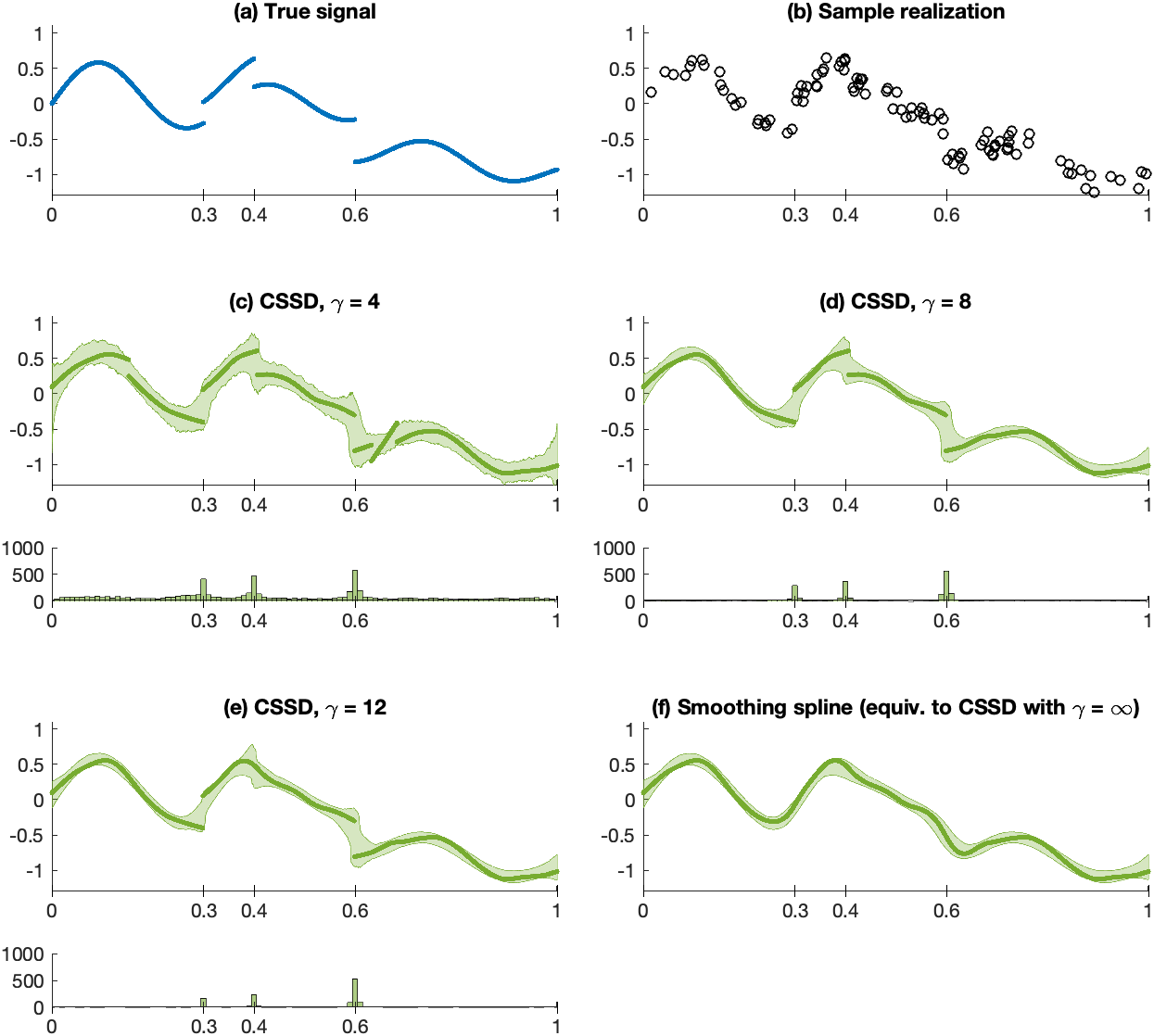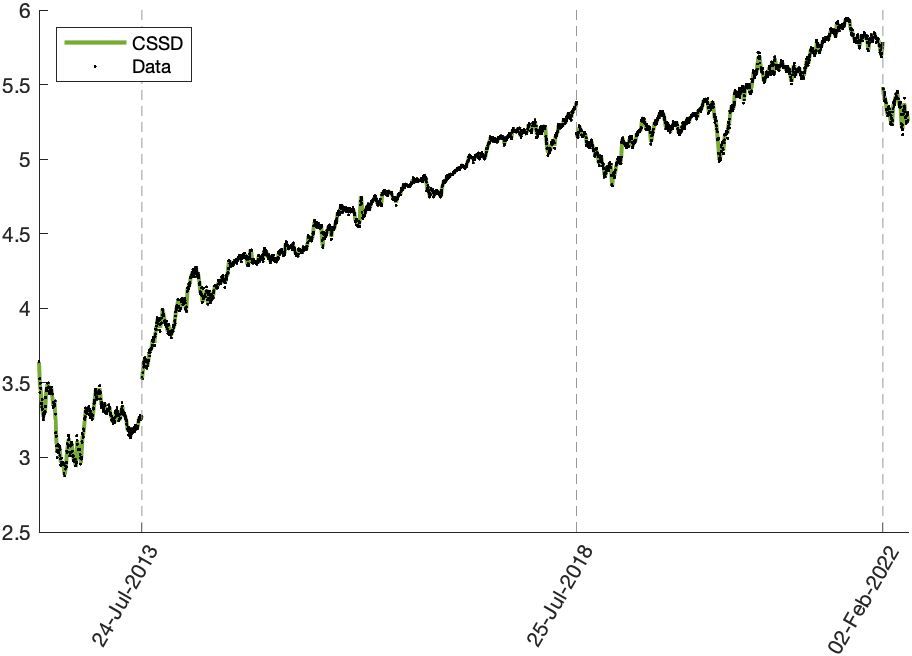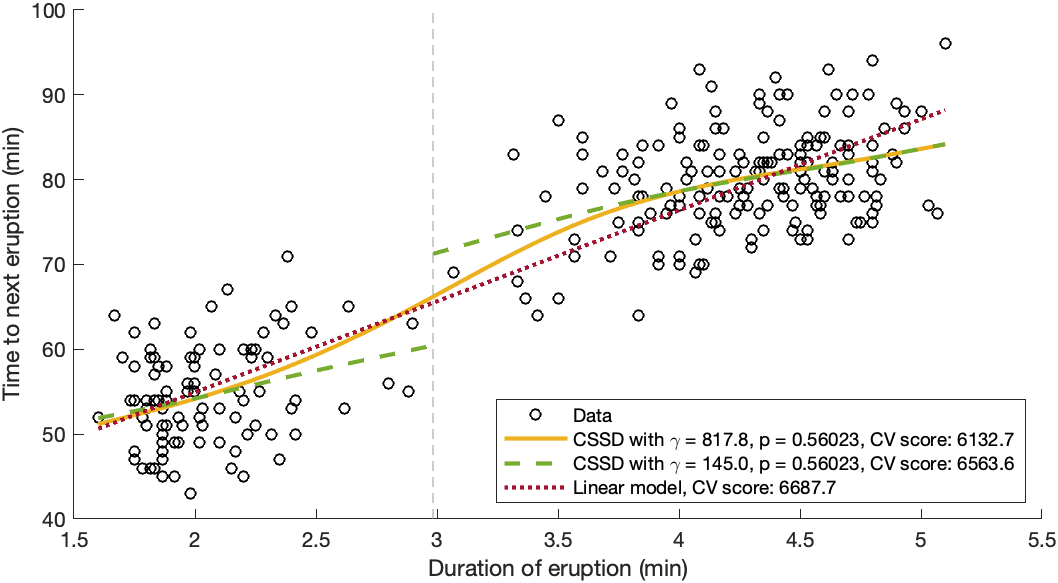## CSSD - Cubic smoothing splines for discontinuous signals

Version 0.9 (258 KB) by
Smoothing splines for discontinuous signals - Fast algorithm and automatic parameter selection

Updated 27 Nov 2022

From GitHub

CSSD - Cubic smoothing splines for discontinuous signals
This is a reference implementation in Matlab for the algorithms described in the paper
Overview of main functionalities
1. cssd.m computes a cubic smoothing spline with discontinuities (CSSD) for data (x,y). It is a solution of the following model of a smoothing spline f with a-priori unknown discontinuities J
2. cssd_cv.m automatically determines values for the model parameters p and γ based on K-fold cross validation.
Quickstart
1. Execute "install_cssd.m" which adds the folder and all subfolders to the Matlab path.
2. Execute any m-file from the demos folder
Examples
Synthetic dataA synthetic signal is sampled at $N = 100$ random data sites $x_i$ and corrupted by zero mean Gaussian noise with standard deviation $0.1.$ The results of the discussed model are shown for $p=0.999$ and different parameters of $\gamma,$ where $\gamma=\infty$ corresponds to classical smoothing splines. The thick lines represent the results of the shown sample realization. The shaded areas depict the $2.5 \%$ to $97.5 \%$ (pointwise) quantiles of $1000$ realizations. The histograms under the plots show the frequency of the detected discontinuity locations over all realizations.Stock dataThe dots represent the logarithm of the closing prices of the Meta stock from May 18, 2012, to May 19, 2022. The curve represents the CSSD with parameters determined by K-fold CV ($p = 0.4702$, $\gamma = 0.0069$). The dashed vertical lines indicate the discontinuities of the CSSD, and the ticks correspond to the date before the discontinuity.Geyser dataFitting a CSSD to the Old Faithful data (circles): If the parameter is selected based on K-fold CV we obtain a result without discontinuities which coincides with a classical smoothing spline (solid curve). Keeping the selected $p$-parameter and lowering the $\gamma$ parameter sufficiently gives a two-phase regression curve (dashed curves) with a breakpoint near $x = 3$ (dashed vertical line), and the two curve segments are nearly linear. Both of the above parameter sets yield better CV-scores than a linear model (dotted line).
Reference
M. Storath, A. Weinmann, "Smoothing splines for discontinuous signals", arXiv:2211.12785, 2022

### Cite As

Martin Storath (2023). CSSD - Cubic smoothing splines for discontinuous signals (https://github.com/mstorath/CSSD/releases/tag/v0.9), GitHub. Retrieved .

Storath, Martin, and Andreas Weinmann. Smoothing Splines for Discontinuous Signals. arXiv, 2022, doi:10.48550/ARXIV.2211.12785.

View more styles
##### MATLAB Release Compatibility
Created with R2022b
Compatible with any release
##### Platform Compatibility
Windows macOS Linux

### Community Treasure Hunt

Find the treasures in MATLAB Central and discover how the community can help you!

Start Hunting!

#### subroutines

Version Published Release Notes
0.9

To view or report issues in this GitHub add-on, visit the GitHub Repository.
To view or report issues in this GitHub add-on, visit the GitHub Repository.